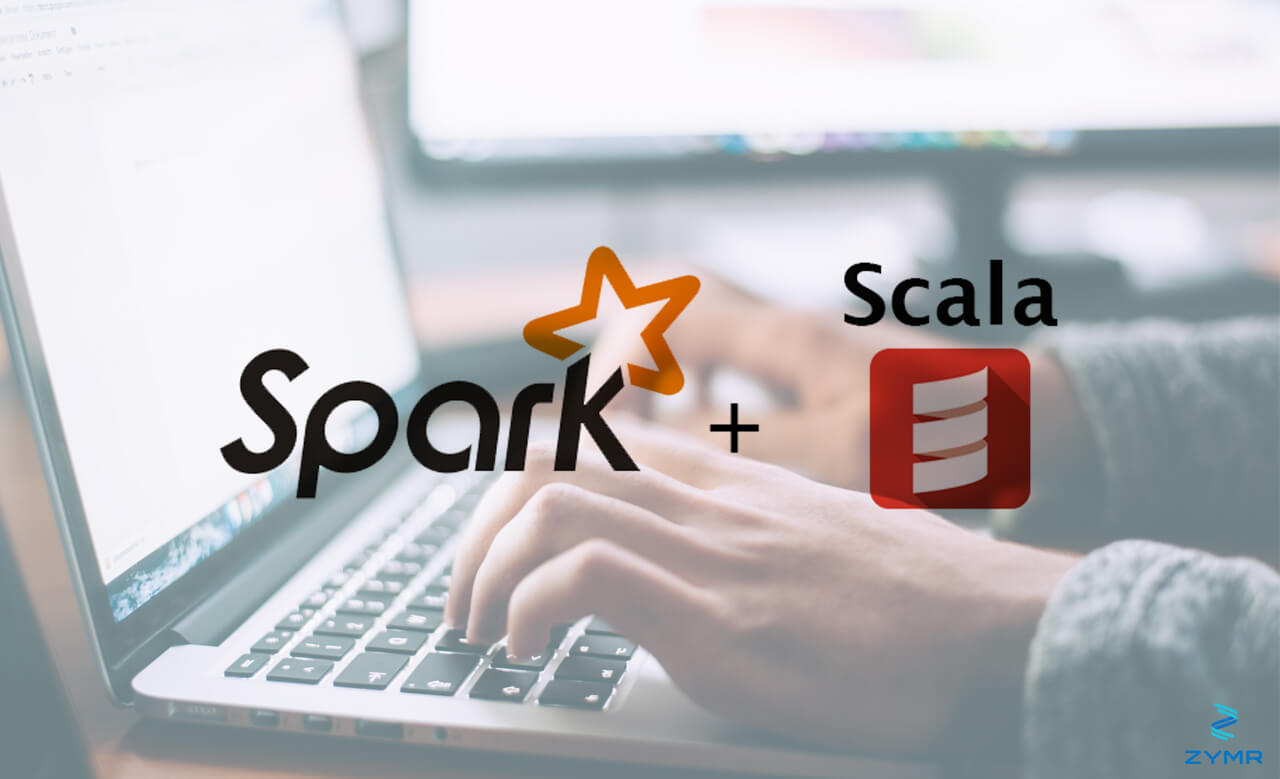Here we provide an example of how to do linear regression using the Spark ML (machine learning) library and Scala. We will do multiple regression example, meaning there is more than one input variable. The goal is to read sample data and then train the Spark linear regression model. From there we can make predicted values given some inputs.

In brief, we want to find an equation that we can use to predict some dependant variable, y, based on some independent variables x1, x2, x3, … . So, the goal is to find the coefficients α, β, γ, … and intercept b to end up with this simple model:

y = α(x1) + β(x2) + γ(x3) + … + ω(xn) + b

Linear Regression Assumptions and the SVM File Format

There are all kinds of rules to check whether the assumption that our data fits the rules of linear regression. For example, there can be no correlation between dependant variables. Also the error of the model is a Gaussian distribution, meaning a standard one. In fact, the error of the model is the average of variance of the errors, meaning the difference between predicted and observed values, which should follow a normal distribution.

We start with the input data shown below. This is a CSV file. To check that we would first plot it and see if visually it appears that there might be some relationship between input and output variables. Of course, we can only do that with one input variable at a time or two as when the number of input variables is > 2 it is not possible to visualize that in a graph.

It does not matter what the columns of data mean, a point which will be illustrated when we convert this to the SVM format. Just know that our dependant variable is in the first column and the remaining columns are independent variables. You would only need to know what the columns are when you want to apply this to whatever problem you are trying to solve. In other words, when you use the Spark ML predict() method or just write down the coefficients and intercept and calculate values based on that.

Input data:

0.84,4.3568,0.83,0.28,4.356839
0.88,5.0000,0.82,0.28,4.357467
0.86,4.3000,0.80,0.28,4.422400
0.86,5.0000,0.80,0.28,4.422400
0.89,4.4259,0.80,0.28,4.425937
0.89,4.4259,0.80,0.28,4.425937
0.89,5.0000,0.79,0.28,4.437922
0.89,4.8000,0.79,0.28,4.448702
0.91,5.0000,0.79,0.28,4.448702

Now we need to convert this to SVM format, as shown below, which is the format used by most statistical software packages for linear regression. Basically the first value is the label (aka index) and the features are an array of features in label:feature format. Here we just use features “1”, “2”, and “3” instead of their description column heading names such as we would have in the spreadsheet we started working with.

0.84 1:4.3568 2:0.83 3:0.28
0.88 1:5.0000 2:0.82 3:0.28
0.86 1:4.3000 2:0.80 3:0.28
0.86 1:5.0000 2:0.80 3:0.28
0.89 1:4.4259 2:0.80 3:0.28
0.89 1:4.4259 2:0.80 3:0.28
0.89 1:5.0000 2:0.79 3:0.28
0.89 1:4.8000 2:0.79 3:0.28
0.91 1:5.0000 2:0.79 3:0.28

Convert CSV File to SVM

This code converts the CSV file to SVM format. The data needs to be put into a Spark Dataframe, which we could do directly. But it is simpler to read in the data, convert it to SVM format, and then use the Spark’s ability to read SVM files directly to convert it to the dataframe that we will use as our training data set.

import scala.io.Source

import java.io._

for (lines var line = lines.split(“,”)
pw.write(line (0) + ” 1:” + line(1) + ” 2:” + line(2) + ” 3:” + line(3) + “\n”)
}

pw.close()

Spark ML Code

We copy the code below directly from the Spark ML documentation.
In order to run this Scala code you would need to start spark-console and paste it in there.

import org.apache.spark.ml.regression.LinearRegression

val lr = new LinearRegression().setMaxIter(10).setRegParam(0.3).setElasticNetParam(0.8)

val lrModel = lr.fit(training)

println(s”Coefficients: \${lrModel.coefficients} Intercept: \${lrModel.intercept}”)

val trainingSummary = lrModel.summary
println(s”numIterations: \${trainingSummary.totalIterations}”)
println(s”objectiveHistory: \${trainingSummary.objectiveHistory.toList}”)
trainingSummary.residuals.show()
println(s”RMSE: \${trainingSummary.rootMeanSquaredError}”)
println(s”r2: \${trainingSummary.r2}”)

Now we ask Spark what intercepts it has found using:

println(s”Coefficients: \${lrModel.coefficients} Intercept: \${lrModel.intercept}”)

It shows these coefficients and intercept. As you can see the 2nd and 3rd coefficients are blank. So the input data we have is either not correlated in the manner that we hoped or we have not satisfied some other condition to apply linear regression.

Coefficients: (3,[],[]) Intercept: 0.8788888888888889

At this point we have we would abandon this model and look for another that better fits the data. Or we might find there is no correlation at all between the input and output data.

This article was authored by Suhas Phartale, who is a Director of Engineering at Zymr.